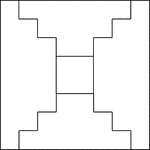### Geometric Block Pattern 58

Geometric pattern for translation and rotation exercises.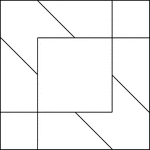### Geometric Block Pattern 59

Geometric pattern for translation and rotation exercises.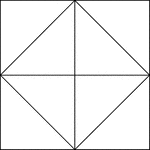### Geometric Block Pattern 6

Geometric pattern for translation and rotation exercises.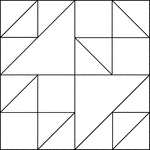### Geometric Block Pattern 60

Geometric pattern for translation and rotation exercises.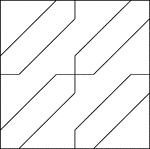### Geometric Block Pattern 61

Geometric pattern for translation and rotation exercises.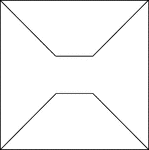### Geometric Block Pattern 62

Geometric pattern for translation and rotation exercises.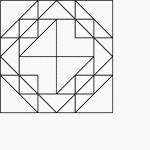### Geometric Block Pattern 63

Geometric pattern for translation and rotation exercises.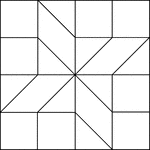### Geometric Block Pattern 64

Geometric pattern for translation and rotation exercises.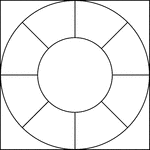### Geometric Block Pattern 66

Geometric pattern for translation and rotation exercises.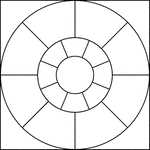### Geometric Block Pattern 67

Geometric pattern for translation and rotation exercises.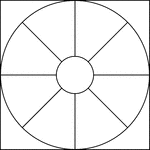### Geometric Block Pattern 68

Geometric pattern for translation and rotation exercises.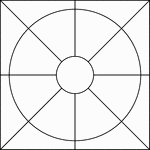### Geometric Block Pattern 69

Geometric pattern for translation and rotation exercises.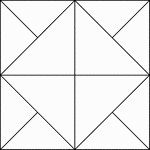### Geometric Block Pattern 7

Geometric pattern for translation and rotation exercises.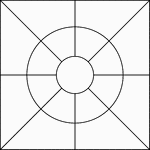### Geometric Block Pattern 70

Geometric pattern for translation and rotation exercises.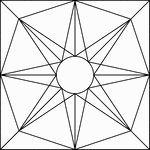### Geometric Block Pattern 71

Geometric pattern for translation and rotation exercises.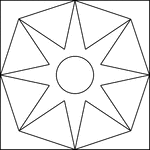### Geometric Block Pattern 72

Geometric pattern for translation and rotation exercises.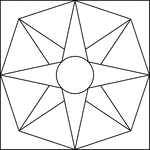### Geometric Block Pattern 73

Geometric pattern for translation and rotation exercises.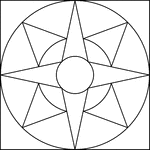### Geometric Block Pattern 74

Geometric pattern for translation and rotation exercises.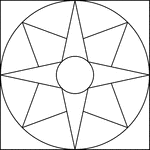### Geometric Block Pattern 75

Geometric pattern for translation and rotation exercises.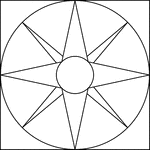### Geometric Block Pattern 76

Geometric pattern for translation and rotation exercises.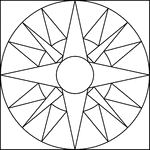### Geometric Block Pattern 77

Geometric pattern for translation and rotation exercises.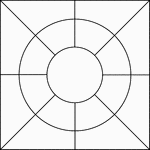### Geometric Block Pattern 78

Geometric pattern for translation and rotation exercises.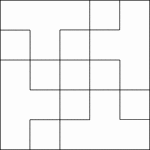### Geometric Block Pattern 79

Geometric pattern for translation and rotation exercises.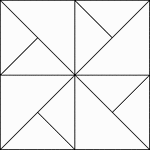### Geometric Block Pattern 8

Geometric pattern for translation and rotation exercises.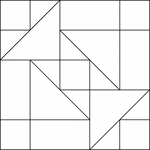### Geometric Block Pattern 80

Geometric pattern for translation and rotation exercises.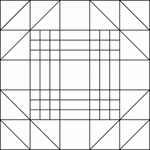### Geometric Block Pattern 81

Geometric pattern for translation and rotation exercises.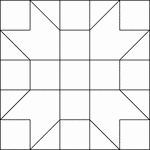### Geometric Block Pattern 82

Geometric pattern for translation and rotation exercises.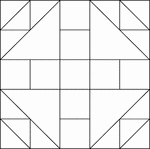### Geometric Block Pattern 83

Geometric pattern for translation and rotation exercises.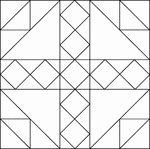### Geometric Block Pattern 84

Geometric pattern for translation and rotation exercises.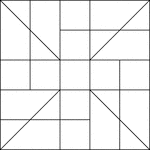### Geometric Block Pattern 85

Geometric pattern for translation and rotation exercises.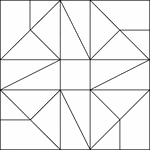### Geometric Block Pattern 86

Geometric pattern for translation and rotation exercises.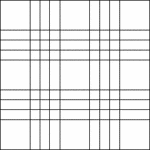### Geometric Block Pattern 87

Geometric pattern for translation and rotation exercises.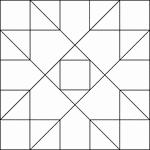### Geometric Block Pattern 88

Geometric pattern for translation and rotation exercises.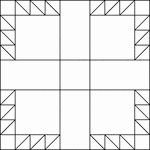### Geometric Block Pattern 89

Geometric pattern for translation and rotation exercises.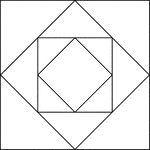### Geometric Block Pattern 9

Geometric pattern for translation and rotation exercises.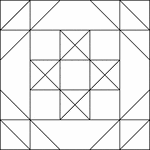### Geometric Block Pattern 90

Geometric pattern for translation and rotation exercises.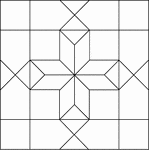### Geometric Block Pattern 91

Geometric pattern for translation and rotation exercises.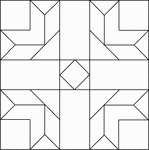### Geometric Block Pattern 92

Geometric pattern for translation and rotation exercises.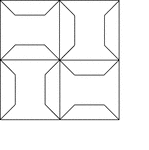### Geometric Block Pattern 93

Geometric pattern for translation and rotation exercises.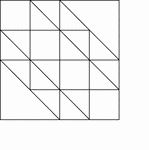### Geometric Block Pattern 94

Geometric pattern for translation and rotation exercises.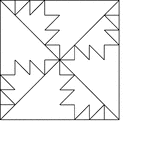### Geometric Block Pattern 95

Geometric pattern for translation and rotation exercises.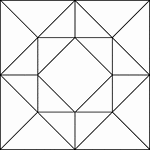### Geometric Block Pattern 96

Geometric pattern for translation and rotation exercises.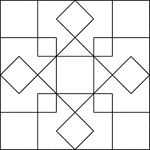### Geometric Block Pattern 97

Geometric pattern for translation and rotation exercises.### Geometric Block Pattern 98

Geometric pattern for translation and rotation exercises.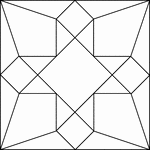### Geometric Block Pattern 99

Geometric pattern for translation and rotation exercises.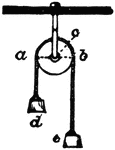### Pulley System

A system of pulleys used to lift an object.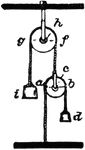### Pulley System

A system of pulleys used to lift an object.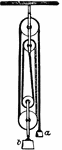### Pulley System

A system of pulleys used to lift an object.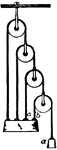### Pulley System

A system of pulleys used to lift an object.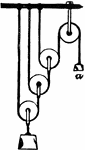### Pulley System

A system of pulleys used to lift an object.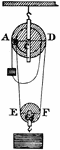### Pulley System

A system of pulleys used to lift an object.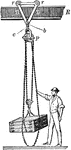### Pulley System

A system of pulleys used to lift an object.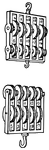### Pulleys - Smeaton's Blocks

"A system of pulleys in two blocks, so arranged that the parts of continuous rope are approximately…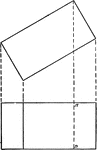### Projections of Rectangular Prism

Projections of a rectangular prism or block.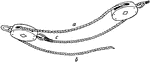### Running Part

"Running-part. Naut., that part of a rope which is hauled upon is called the hauling-part (b), the part…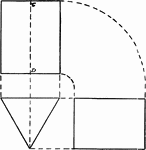### Projections of Triangular Prism

Projections of a triangular prism or block.### Projections of Triangular Prism With Hole

Projections of a triangular prism or block with a square hole.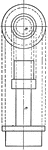### Projection of Turned Block

Projection of a turned block.